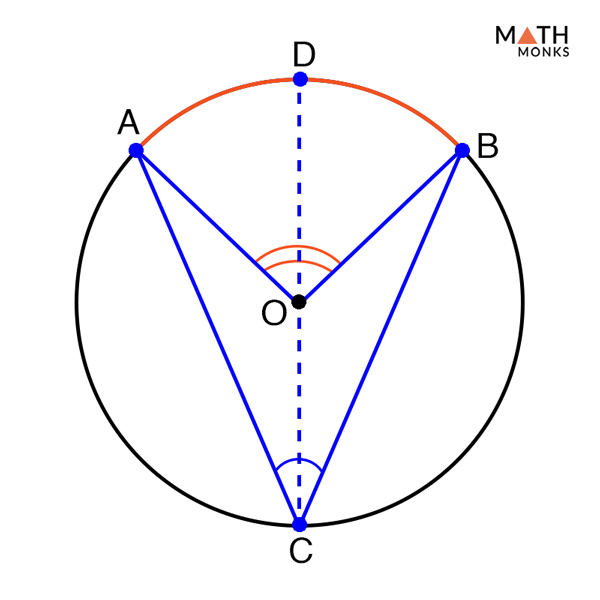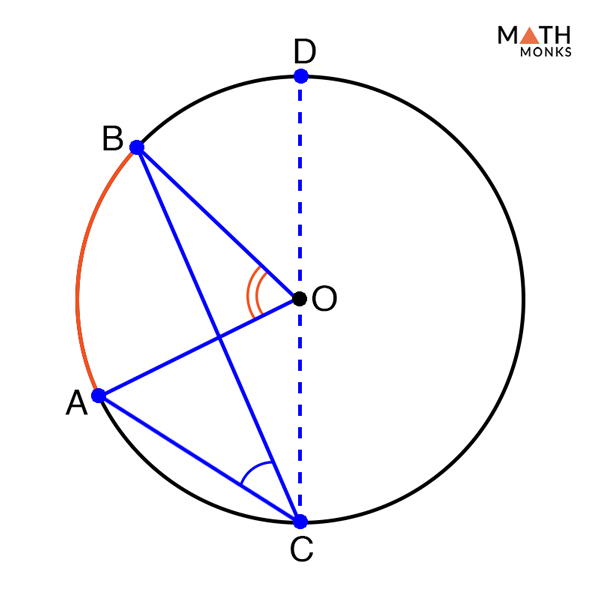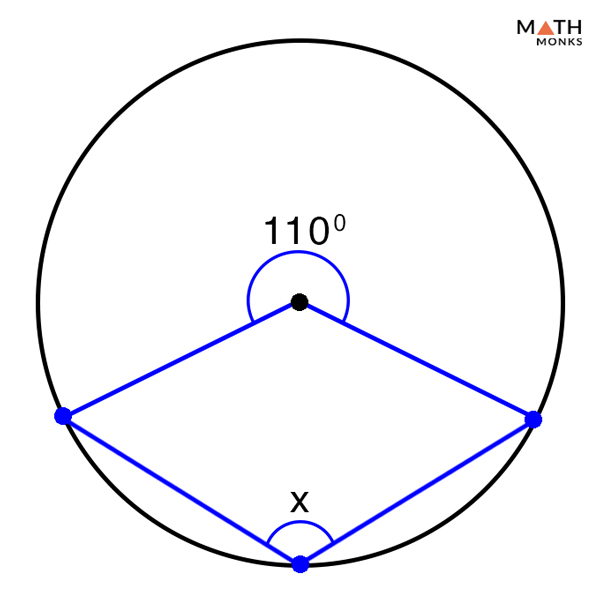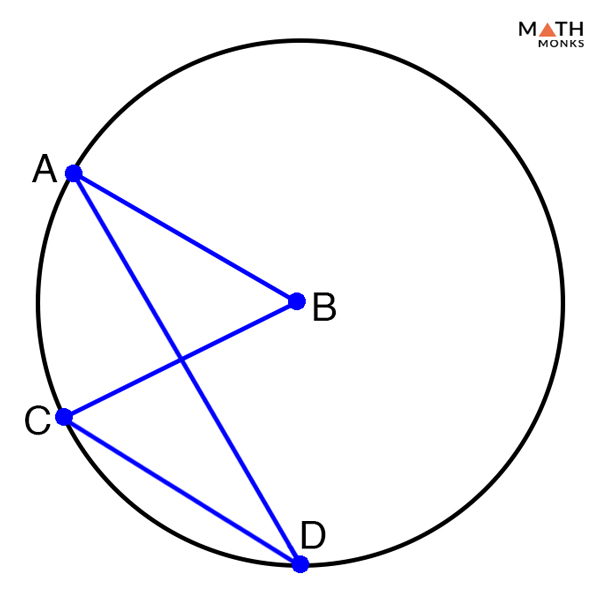# Inscribed Angle

## What is an Inscribed Angle

An inscribed angle is an angle whose vertex lies on the circumference of a circle while its two sides are chords of the same circle. The arc formed by the inscribed angle is called the intercepted arc.

The above figure shows a circle with center O having an inscribed angle, ∠ABC. The two arms AB and BC are necessarily two cords of the same circle, while AC is the intercepted arc.

## Inscribed Angle Theorem

In order to prove this theorem, we need to consider three separate cases. Each of them will differ based on where the center lies in comparison to the inscribed angle.

Case 1: Prove Inscribed Angle Theorem when the inscribed angle is between a chord and the diameter of a circle

To prove:

∠OCB = ½ ∠AOB or 2∠OCB = ∠AOBProof:

Let ∠ACB be the inscribed angle formed between the chord ‘CB’ and the diameter ‘AC’ of the circle
∠AOB = 2∠OCB
In the given figure,
△OBC is an isosceles triangle, where OC = OB = radius of the circle
Hence, ∠OCB = ∠CBO = inscribed angle
Now, the diameter AD is a straight line,
So, ∠BOC = 180° – ∠AOB…. (1)
By triangle sum theorem,
∠OCB + ∠CBO +∠BOC = 180°
Now, ∠OCB = ∠CBO (Congruent angles in the isosceles △OBC) and from (1) we get,
2∠OCB + (180° – ∠AOB) = 180°
Subtract 180 on both sides, we get,
2∠OCB + 180° – ∠AOB = 180°
2∠OCB – ∠AOB = 0
2∠OCB = ∠AOB
Hence Proved

Case 2: Prove Inscribed Angle Theorem when the diameter is between the two chords forming the inscribed circle

To prove:

∠AOB = 2∠ACBProof:

Let the diameter (shown by imaginary line) bisects:
∠ACB into ∠ACO and ∠BCO
∠ACB into ∠AOD and ∠BOD
Thus,
∠ACO + ∠BCO = ∠ACB…… (1)
∠AOD + ∠BOD = ∠AOB…… (2)
Now, from the first case above, we know,
2∠ACO = ∠AOD…. (3)
2∠BCO = ∠BOD….. (4)
Adding (3) and (4), we get,
∠AOD + ∠BOD = 2∠ACO + 2∠BCO
∠AOD + ∠BOD = 2(∠ACO + ∠BCO)
Now, from (1) and (2), we get,
∠AOB = 2∠ACB
Hence Proved

Case 3: Prove Inscribed Angle Theorem when the diameter is outside the two chords forming the inscribed angle

To prove:

∠AOB = 2∠ACBProof:

Since, 2∠BCO = ∠BOD
2(∠BCO + ∠ACB) = ∠AOB + ∠BOD
2∠BCO + 2∠ACB = ∠AOB + ∠BOD
Now, since 2∠BCO = ∠BOD
2∠ACB = ∠AOB
Hence Proved

## Formula

### How to Solve an Inscribed Angle

Inscribed angle of a circle can be determined if its corresponding central angle is known by using the formula derived from the inscribed angle theorem given below:

Inscribed Angle = Central angle/2

Let us solve some problems to understand the concepts better.Solve the missing angle x in the diagram given below.

Solution:

As we know,
According to the inscribed angle theorem, the measure of the inscribed angle is half the measure of the central angle,
Given,
Central angle = 110°
Thus,
Inscribed angle = 110°/2 = 55°Given that ∠ABC = (4x + 20)° and ∠CDA = 20°. Find the value of x.

Solution:

As we know,
According to the inscribed angle theorem, the measure of the inscribed angle is half the measure of the central angle,
∠ABC = 2∠CDA
Hence,
∠ABC =2 x 20° = 40°
Now,
(4x + 20)° = 40°
4x = 40°- 20°
x =20°/4 = 5°